How Cheenta works to ensure student success?
Explore the Back-Story

# Men and Job Problem | Tomato Question 2 | ISI EntranceThis is a problem from TOMATO Problem number 2, useful for ISI and CMI entrance exam based on Men and Job.

Problem:

If m men can do a job in d days, then the number of days in which m+r men can do the job is
(A) d+r; (B)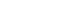; (C)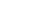; (D);

Discussion:

If m men can do a job in d days,
Then m men does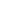job in 1 day
Then 1 man does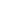job in 1 day
Then m+r men does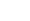job in 1 day
Then m+r men does 1 jobdays.

Hence answer isdays (D)

## Chatuspathi:

• What is this topic: Arithmetic
• What are some of the associated concepts: Unitary Method
• Where can learn these topics: Cheenta I.S.I. & C.M.I. course, discusses these topics in the ‘Arithmetic’ module.
• Book Suggestions: Arithmetic by K.C. Nag

This is a problem from TOMATO Problem number 2, useful for ISI and CMI entrance exam based on Men and Job.

Problem:

If m men can do a job in d days, then the number of days in which m+r men can do the job is
(A) d+r; (B); (C); (D);

Discussion:

If m men can do a job in d days,
Then m men doesjob in 1 day
Then 1 man doesjob in 1 day
Then m+r men doesjob in 1 day
Then m+r men does 1 jobdays.

Hence answer isdays (D)

## Chatuspathi:

• What is this topic: Arithmetic
• What are some of the associated concepts: Unitary Method
• Where can learn these topics: Cheenta I.S.I. & C.M.I. course, discusses these topics in the ‘Arithmetic’ module.
• Book Suggestions: Arithmetic by K.C. Nag

This site uses Akismet to reduce spam. Learn how your comment data is processed.

### Knowledge Partner